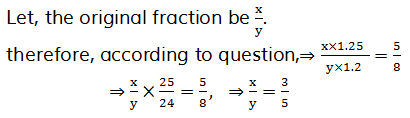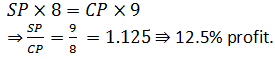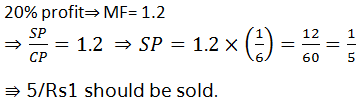# Percentage Concepts, Formulae & Tricks

## Multiplying Factor:

### 1. When a quantity is increased:

Suppose, we have to increase 100 by 20%,
Then, Final value = 100+20% of 100
= 100+1/5 ✕100= 100+20= 120
Here, Multiplying factor(M.F.) =1.2

#### Example:

Price of egg increase by 10% in December from previous month & again increases by 20% in January from December. Find the new price of the egg if the old price was 5R?
Solution: For 10% increase, M.F.1= 1.1
for 20% increase, M.F.2= 1.2
Therefore, New price= 5× 1.1× 1.2 = 5.5×1.2=6.60 R

### 2. When quantity is decreased:

Suppose, we have to decrease 100 by 30%,
Then, final value= 100-30% of 100
= 100-30= 70
Here, Multiplying factor (M.F.) = 0.7

#### Example:

There is 10%, 15% & 20% depreciation in the value of mobile phone in 1st, 2nd & 3rd month after sale if the price at beginning was 10,000R, then the price of mobile after 3rd month will be:-
Solution: Final Price= Original Price×MF1×MF2×MF3
⇒ = 10000×0.9×0.85×0.8 = 6120 D

### NOTE:

10% increase ⇒ MF=1.1
10% decrease⇒ MF=0.9
5%increase ⇒MF=1.05
5%decrease ⇒ MF=0.95

### Example1:

If the numerator of a fraction is increased by 25% and the denominator is increased by 20%, the resultant fraction is 5/8. What was the original fraction?
Solution:### Example2:

The population of a town is increased by 9.09% in the first year, decreased by 11.11% in 2nd year & increased by 14.28% in the 3rd year. Find the present population if after 3 years the population will become 512000.
Solution:#### Note### Example1.

If selling price of 8 items is equal to the cost price of 9. Find percentage profit/loss?
Solution:### Example2:

If by selling 8 items one makes a loss of equal to Cost price of 2 items. Find percentage loss?
Solution:

### Example3:

How many oranges should be sold for Rs1 if they were purchased at rate of 6/Rs1 and a profit of 20% is desired.
Solution:### Example4:

If equal amount of Rupees were spent on two lots of bananas. One at a rate of Rs12/dozen and other at Rs18/dozen. If all the bananas are sold at the rate of Rs15/dozen. What is the percentage of profit/loss?
Solution:

## Faulty Balance:

### Example1:

A milkman mixes 100 liter of water with every 800lit. Of milk and sells at a mark-up of 11.11%. Find the percentage profit?
Solution:### Example2:

A meter scale is to measure 90cm. However, it expands 20% in summer. A trader sells at a mark-up of 10%, but he doesn’t know that the whole seller from where he has bought, measure 80cm only. Find percentage profit/loss of trader?
Solution: